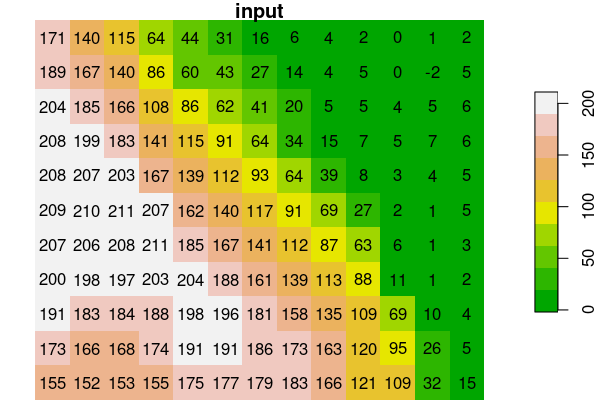## Package `starsExtra`

R package `starsExtra` provides several miscellaneous functions for working with `stars` objects, mainly single-band rasters. Currently includes functions for:

• Focal filtering
• Detrending of Digital Elevation Models
• Calculating flow length
• Calculating the Convergence Index
• Calculating topographic slope
• Calculating topographic aspect

## Installation

CRAN version:

``install.packages("starsExtra")``

GitHub version:

``````install.packages("remotes")
remotes::install_github("michaeldorman/starsExtra")``````

## Usage

Once installed, the library can be loaded as follows:

``````library(starsExtra)
#> Linking to GEOS 3.8.0, GDAL 3.0.4, PROJ 6.3.1

## Examples

### Focal filter: aggregation functions

``````data(dem)
dem_mean3 = focal2(dem, matrix(1, 3, 3), "mean", na.rm = TRUE)
dem_sum3 = focal2(dem, matrix(1, 3, 3), "sum", na.rm = TRUE)
dem_min3 = focal2(dem, matrix(1, 3, 3), "min", na.rm = TRUE)
dem_max3 = focal2(dem, matrix(1, 3, 3), "max", na.rm = TRUE)``````
``````plot(dem, main = "input", text_values = TRUE, breaks = "equal", col = terrain.colors(10))
plot(dem, col = rep(NA, 3), key.pos = NULL, main = "")
plot(round(dem_mean3, 1), main = "mean (k=3)", text_values = TRUE, breaks = "equal", col = terrain.colors(10))
plot(dem_sum3, main = "sum (k=3)", text_values = TRUE, breaks = "equal", col = terrain.colors(10))
plot(dem_min3, main = "min (k=3)", text_values = TRUE, breaks = "equal", col = terrain.colors(10))
plot(dem_max3, main = "max (k=3)", text_values = TRUE, breaks = "equal", col = terrain.colors(10))``````